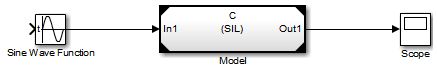## Choose a SIL or PIL Approach

Consider a top model that consists of components `A`, `B`, `C`, and `D`:

• `A` and `B` are existing components for which code has previously been generated and tested.

• `C`, a referenced model, and `D`, a subsystem, are new components.With software-in-the-loop (SIL) and processor-in-the-loop (PIL) simulations, you can use the following approaches to numerical equivalence testing:

For some forms of testing, you require a test harness model. The test harness model:

• Generates test vectors or stimulus inputs that feed the block under test.

• Makes it possible for you to observe or capture output from the block.

The following example shows a simple test harness model.The block under test is a Model block. The Sine Wave block generates the input for the Model block. Through the Scope block, you can observe the output from the Model block. For information about creating and using test harnesses, see:

### Test Top-Model Code

To test code generated from the top-model components together (`A`, `B`, `C`, and `D`), you can use top-model SIL/PIL or Model block SIL/PIL.

• Top-model SIL/PIL:

1. Create test vectors or stimulus inputs in the MATLAB workspace.

2. Run the top model in normal, SIL, and PIL simulation modes. The software loads the test vectors or stimulus inputs from the MATLAB® workspace.

3. For each simulation mode, observe or capture outputs.

4. Verify numerical equivalence by comparing normal outputs against SIL and PIL outputs.

• Model block SIL/PIL:

1. Create a Model block that contains the top-model components.

2. Insert the Model block in a simulation model, for example, your test harness model.

3. Run simulations, switching the Model block between normal, SIL, and PIL modes. For the SIL and PIL simulation modes, set the Code interface Model block parameter to ```Top model```.

4. Verify numerical equivalence by comparing normal outputs against SIL and PIL outputs.

### Test Referenced Model Code

To test code generated from the component `C` as part of a model reference hierarchy, use the Model block SIL/PIL approach:

• Insert the Model block `C` in a simulation model, for example, your test harness model.

• Run simulations, switching the Model block between normal, SIL, and PIL modes. For the SIL and PIL simulation modes, set the Code interface Model block parameter to ```Model reference```.

• Verify numerical equivalence by comparing normal outputs against SIL and PIL outputs.

### Test Subsystem Code

To test code generated from the subsystem `D`, use the SIL or PIL block approach:

1. Insert the subsystem in a simulation model, for example, your test harness model.

2. Run a normal mode simulation, capturing the outputs.

3. Create a SIL or PIL block from the subsystem.

4. In the model, replace the subsystem with the SIL or PIL block.

5. Run a simulation of the model, capturing the outputs.

6. Verify numerical equivalence by comparing normal mode subsystem outputs against SIL or PIL block outputs.

### Summary

Simulation TypeComponent From Which Code Is Generated StepsGenerated Code InterfaceTest Signal Source
Top-model SIL/PILTop model

In SIL/PIL Manager:

1. Select SIL/PIL Simulation Only mode.

2. In System Under Test, select `Top model`.

StandaloneMATLAB workspace
Model block SIL/PILModel referenced by Model block

1. In SIL/PIL Manager:

1. Select SIL/PIL Simulation Only mode.

2. In System Under Test, select ```Model blocks in SIL/PIL mode```.

2. In Model blocks, set Simulation mode to ```Software-in-the-loop (SIL)``` or ```Processor-in-the-loop (PIL)```.

Determined by Model block parameter Code interface: standalone or model reference.Simulation model, for example, test harness model
SIL or PIL blockSubsystemManual block substitutionStandaloneSimulation model, for example, test harness model.